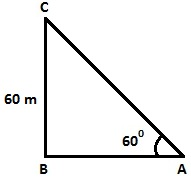# A kite is flying at a height of 60 m above the ground. The string attached to the kite is temporarily tied to a point on the ground. The inclination of the string with the ground is 60°. Find the length of the string, assuming that there is no slack in the string.

From the given data, the figure isLet BC = Height of the kite from the ground, BC = 60 m

AC = Inclined length of the string from the ground and A is the point where string of the kite is tied.

To Find: Length of the string from the ground i.e. the value of AC

sin 60° = BC/AC

⇒ √3/2 = 60/AC

⇒ AC = 40√3 m

Thus, the length of the string from the ground is 40√3 m.